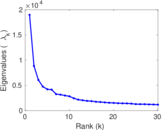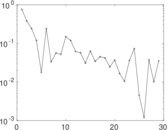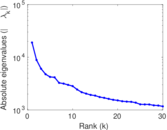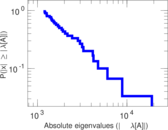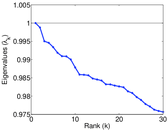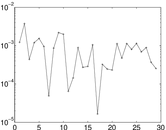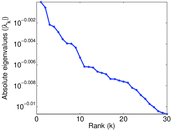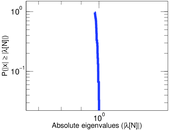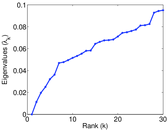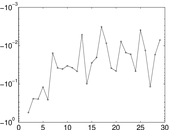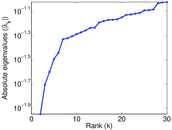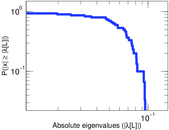# vi.sualize.us user–tag

This is the bipartite picture tagging network of vi.sualize.us. Left nodes represent users and right nodes represent tags. An edge connects a user and a tag he has used.

 Code `Vut` Internal name `pics_ut` Name vi.sualize.us user–tag Data source http://vi.sualize.us/ AvailabilityDataset is available for download Consistency checkDataset passed all tests Category Interaction network Node meaning User, tag Edge meaning Assignment Network formatBipartite, undirected Edge typeUnweighted, multiple edges

## Statistics

 Size n = 99,157 Left size n1 = 17,122 Right size n2 = 82,035 Volume m = 2,298,816 Unique edge count m̿ = 449,503 Wedge count s = 211,070,075 Claw count z = 290,844,387,003 Cross count x = 596,462,431,865,815 Square count q = 519,071,489 4-Tour count T4 = 4,997,987,030 Maximum degree dmax = 405,429 Maximum left degree d1max = 46,571 Maximum right degree d2max = 405,429 Average degree d = 46.367 2 Average left degree d1 = 134.261 Average right degree d2 = 28.022 4 Fill p = 0.000 320 021 Average edge multiplicity m̃ = 5.114 13 Size of LCC N = 98,486 Diameter δ = 12 50-Percentile effective diameter δ0.5 = 3.453 47 90-Percentile effective diameter δ0.9 = 4.781 47 Median distance δM = 4 Mean distance δm = 4.001 17 Gini coefficient G = 0.940 143 Balanced inequality ratio P = 0.082 228 4 Left balanced inequality ratio P1 = 0.148 075 Right balanced inequality ratio P2 = 0.083 396 4 Power law exponent γ = 2.439 72 Tail power law exponent γt = 1.701 00 Degree assortativity ρ = −0.106 507 Degree assortativity p-value pρ = 0.000 00 Spectral norm α = 18,991.5 Algebraic connectivity a = 0.011 268 9 Spectral separation |λ1[A] / λ2[A]| = 2.145 22 Controllability C = 76,829 Relative controllability Cr = 0.774 822

## Plots

### Fruchterman–Reingold graph drawing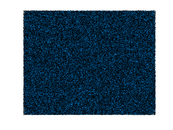### Degree distribution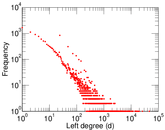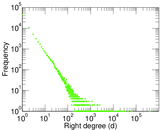### Cumulative degree distribution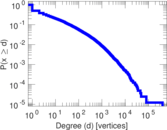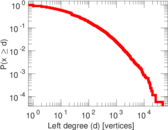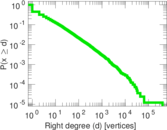### Lorenz curve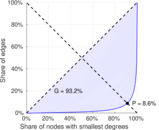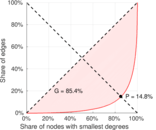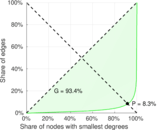### Spectral distribution of the adjacency matrix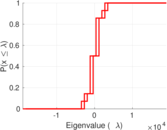### Spectral distribution of the normalized adjacency matrix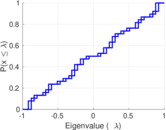### Spectral distribution of the Laplacian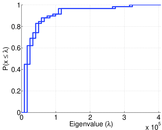### Spectral graph drawing based on the adjacency matrix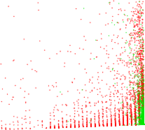### Spectral graph drawing based on the Laplacian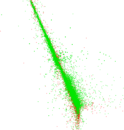### Spectral graph drawing based on the normalized adjacency matrix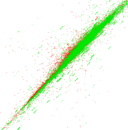### Degree assortativity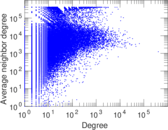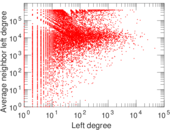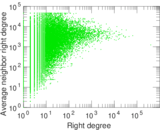### Zipf plot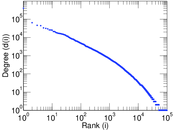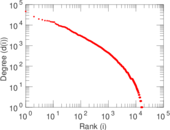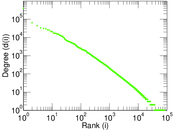### Hop distribution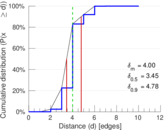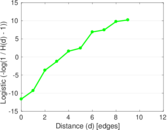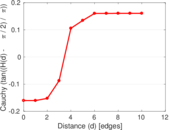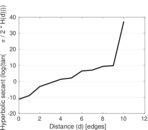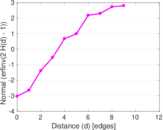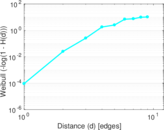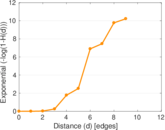### Edge weight/multiplicity distribution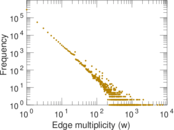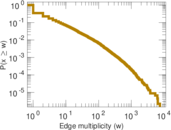### Matrix decompositions plots